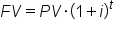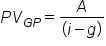# Additional Detail on Present and Future Values

Author: Sophia Tutorial
##### Description:

Determine the effect of interest rates on present and future value.

(more)### Developing Effective Teams

*No strings attached. This college course is 100% free and is worth 1 semester credit.

37 Sophia partners guarantee credit transfer.

299 Institutions have accepted or given pre-approval for credit transfer.

* The American Council on Education's College Credit Recommendation Service (ACE Credit®) has evaluated and recommended college credit for 32 of Sophia’s online courses. Many different colleges and universities consider ACE CREDIT recommendations in determining the applicability to their course and degree programs.

Tutorial

what's covered
In this lesson, you will learn about the effect of interest rates on present and future values. Specifically, this lesson will cover:
1. The Relationship Between Present and Future Value
2. Calculating Perpetuities

## 1. The Relationship Between Present and Future Value

The future value determines what a sum of money will be worth at a period in the future, given various rates of interest, compounding periods, and time. The FV is calculated by multiplying the present value by the accumulation function. The value does not include corrections for inflation or other factors that affect the true value of money in the future. The process of finding the FV is often called capitalization.

On the other hand, the present value (PV) is the value on a given date of a payment or series of payments made at other times. The process of finding the PV from the FV is called discounting.

PV and FV are related, as reflected in the following compounding interest formula.

formula

Compound Interesthint
Simple interest has n multiplied by i, instead of as the exponent. However, since it is rather rare to use simple interest, the compounding interest formula is the important one.

PV and FV vary directly; when one increases, the other increases, assuming that the interest rate and number of periods remain constant.

The interest rate (or discount rate) and the number of periods are the two other variables that affect the FV and PV. The higher the interest rate, the lower the PV and the higher the FV. The same relationships apply for the number of periods. The more time that passes, or the more interest accrued per period, the higher the FV will be if the PV is constant, and vice versa.

The formula implicitly assumes that there is only a single payment. If there are multiple payments, the PV is the sum of the present values of each payment and the FV is the sum of the future values of each payment.

term to know

Capitalization
The process of finding the future value of a sum by evaluating the present value.
Discounting
The process of finding the present value using the discount rate.

## 2. Calculating Perpetuities

A perpetuity is a unique type of annuity. Whereas an annuity has a specified end, a perpetuity is a stream of cash flow payments that has no end – it continues forever. Essentially, they are ordinary annuities, but have no end date. There aren’t many actual perpetuities, but the United Kingdom has issued them in the past.

Since there is no end date, the annuity formulas we have explored don’t apply here. There is no end date, so there is no future value formula. To find the FV of a perpetuity would require setting a number of periods which would mean that the perpetuity up to that point can be treated as an ordinary annuity.

There is, however, a PV formula for perpetuities. The PV is simply the payment size (A) divided by the interest rate (r). Notice that there is no n, or number of periods. More accurately, it is what results when you take the limit of the ordinary annuity PV formula as n → ∞.

It is also possible that an annuity has payments that grow at a certain rate per period. The rate at which the payments change is fittingly called the growth rate (g). The PV of a growing perpetuity is represented with the following formula:

formula

PV of a Growing PerpetuityIt is essentially the same except that the growth rate is subtracted from the interest rate. Another way to think about it is that for a normal perpetuity, the growth rate is just 0, so the formula boils down to the payment size divided by r.

term to know

Growth Rate
The percentage by which the payments grow each period.
summary
In this lesson, you learned more about the relationship between present value and future value, which are directly related. However, the difference between the two is affected by the interest rate and the number of periods. Calculating perpetuities, or payment streams that continue indefinitely, is different from other annuities. This involves knowing the payment size, the interest rate, and the growth rate (in the case of growing perpetuities).

Best of luck in your learning!

Source: THIS CONTENT HAS BEEN ADAPTED FROM LUMEN LEARNING'S “Additional Detail on Present and Future Values” TUTORIAL.

Terms to Know
Capitalization

The process of finding the future value of a sum by evaluating the present value.

Discounting

The process of finding the present value using the discount rate.

Growth Rate

The percentage by which the payments grow each period.

Formulas to Know
Compound InterestPV of a Growing PerpetuityRating